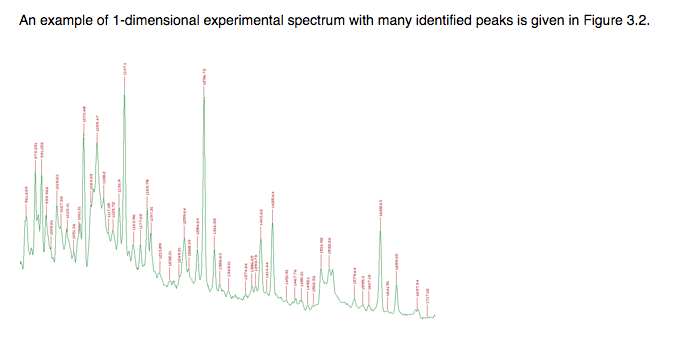# Add labels to peaks found by TSpectrum on histogram

Hi,

I would like to know how to add labels to peaks identified by the TSpectrum class.
Something similar to what I found here:

https://root.cern.ch/root/html534/guides/spectrum/Spectrum.html#dimensional-spectra-3

Thanks,
Laura_ROOT Version: ROOT 6.12/06

_Platform: OS X Yosemite 10.10.5

_Compiler: Apple LLVM version 7.0.0 (clang-700.1.76)
Target: x86_64-apple-darwin14.5.0

Hi Laura,

there is no built-in functionality to do this. You can either trigger the drawing of the bin content for each bin (with the `text` drawing option for your histogram) or (which I think is better) you can draw it as a text object next to every identified peak. Since you fit the peaks a gaussian anyway, you can just draw the “constant” at positions returned by `s->GetPositionX()`.

Could you be more explicit and tell me how to do this?
Thanks!

``````Int_t npeaks = 30;
double fpeaks(double *x, double *par) {
double result = par + par*x;
for (Int_t p=0;p<npeaks;p++) {
double norm  = par[3*p+2]; // "height" or "area"
double mean  = par[3*p+3];
double sigma = par[3*p+4];
result += norm*TMath::Gaus(x,mean,sigma);
}
return result;
}

void peakstext(Int_t np=10) {
npeaks = TMath::Abs(np);
TH1F *h = new TH1F("h","test",500,0,1000);
// Generate n peaks at random
double par;
par = 0.8;
par = -0.6/1000;

for (int p=0;p<npeaks;p++) {
par[3*p+2] = 1; // "height"
par[3*p+3] = 10+gRandom->Rndm()*980; // "mean"
par[3*p+4] = 3+2*gRandom->Rndm(); // "sigma"
}

TF1 *f = new TF1("f",fpeaks,0,1000,2+3*npeaks);
f->SetNpx(1000);
f->SetParameters(par);
auto *c1 = new TCanvas();

h->FillRandom("f",200000);

TSpectrum *s = new TSpectrum(2*npeaks);
Int_t nfound = s->Search(h,2,"",0.10);

TList *functions = h->GetListOfFunctions();
TPolyMarker *pm = (TPolyMarker*)functions->FindObject("TPolyMarker");
double *xp = pm->GetX();
double *yp = pm->GetY();
int      n = pm->GetN();
for (int i = 1; i<n; i++) {
auto t = new TText(xp[i],yp[i],Form("%g", yp[i]));
t->Draw();
}
}

``````
1 Like

Great! Thanks a lot.

Of course some polishing is needed to nicely display de text (font, angle, size position, size …) , and choose the right text. But you have the idea.

This topic was automatically closed 14 days after the last reply. New replies are no longer allowed.